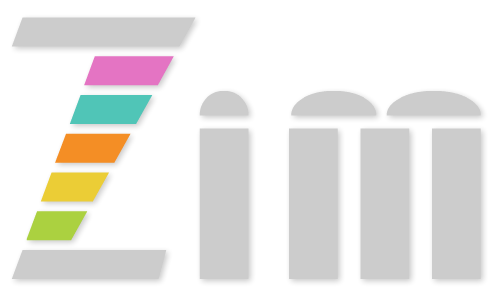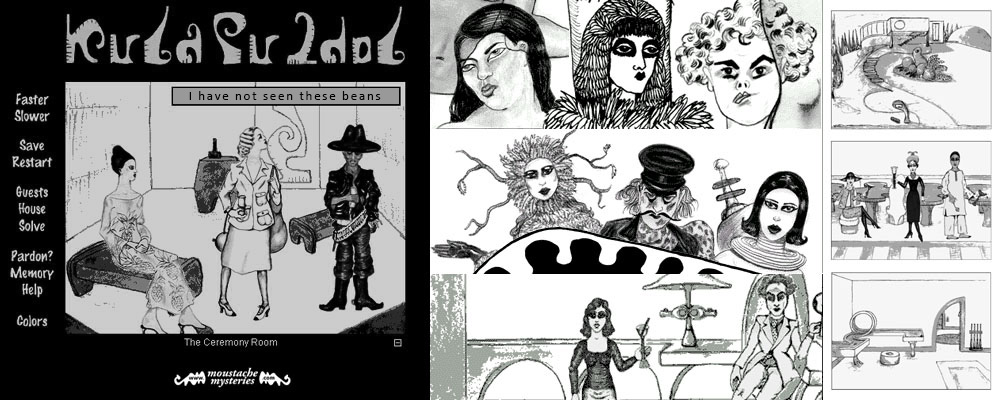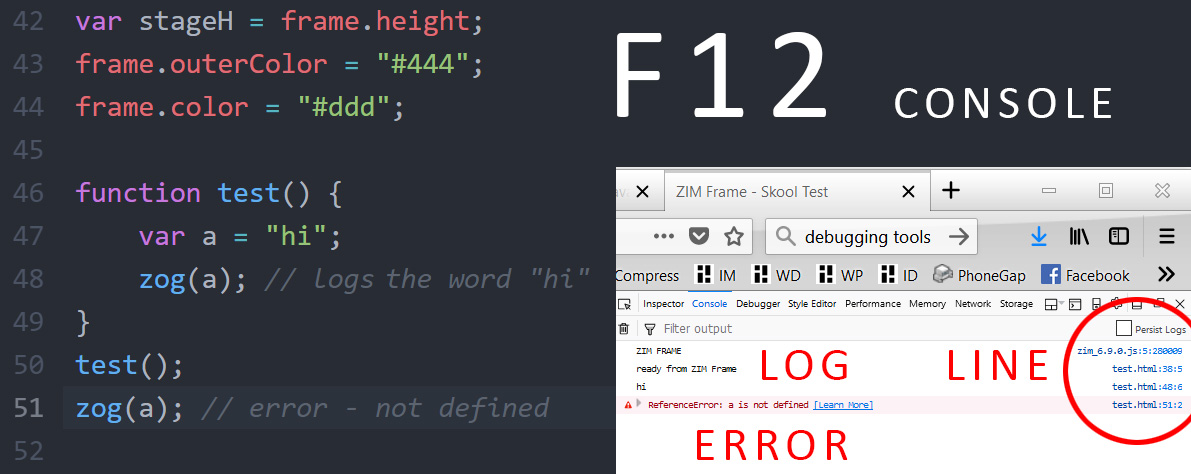# ZIM - Code and Learn Coding with ZIM for JavaScript and HTML Canvas with CreateJS## Practice with ZIM CODE see the Learn Page for tutorials

 ALL LESSONS# Lesson 06 - Conditionals and Debugging

Creative Coding Videos are above at right - they are a few versions behind the current ZIM.
Differences are that time is now in seconds not milliseconds and ES6 is now used not ES5.

## Theory

A very important part of coding is logic. Logic guides what happens and when to create the flow of an application. A conditional is what we use to apply logic. It is much easier than it sounds as it is simply an if statement. For example:

if the hour is greater than eight then eat breakfast ;-)

We combine conditionals with random numbers to make what we code more life-like. This gives us odds which are important in games, artificial intelligence, generative art, etc.

The Dan Zen Museum Coding Tour gives a description of how conditionals with random numbers lets us build a mystery with secret keeping, and different answers depending on who is in the room, etc.Character Drawings by Greg Rennick - backgrounds and production by Dan Zen

Along with conditionals, we will take a look at debugging. A bug is the cute term for when the code does not work. An actual bug (a moth) short curcuited a computer way back in 1945. These days, it means we have made a mistake in our code. We will list a variety of ways for us to find and fix the mistake.

Let's take a look at REFERENCE and PRACTICE sections for conditionals and debugging!

## Reference

Conditionals ► What IZ a Conditional?
A conditional lets you test if something is true. We use an if statement and put an expression to test inside ( ). If the expression evaluates to true, then we do the block of code inside the { }.

01
```// a simple if statement
let x = 10;
if (x > 5) {
zog("win!");
}
```

If what is inside the ( ) is true, then do what is inside the { }.

The ( ) have the expression x > 5 which is true because x is 10. So we run the code in the { } to log "win!" to the console. If x were 2 then "win!" would NOT be logged to the console.

Note the similarities between conditionals, functions and loops:
if ( ) { }
function ( ) { }
for ( ) { }

Operators ► What IZ an Operator?
We often use Boolean, comparison and logical operators inside the ( ) of the if statement. These are:

#### Operators

• > greater than
• >= greater than or equal
• < less than
• <= less than or equal
• == equal (note the two equal signs)
• === strict equal (and types equal)
• ! not
• || or
• && and
• () group

02
```// a BROKEN if statement
let x = 10;
if (x = 5) {
// this code will always run
// because we are ASSIGNING 5 to x
// and this results in testing 5
// any number is considered true except for 0
// we wanted the == comparison operator
// not the = assignment operator
zog("oops!");
}
```

03
```// equal and strict equal
let x = 10; // Number
let y = "10"; // String
if (x == y) {
// this code runs as JavaScript converts types to same type
}
if (x === y) {
// this code does not run as the types are different
}
```

04
```// Multiple Operators
let x = 10;
if (x > 5 && x < 20) {
zog ("inbetween!");
}

if (!(x == 3 || x == 6 || x == 9)) {
zog ("not pregant threes!");
}
```

Boolean
A Boolean is an object with a value of true or false. When tested, any expression evaluates to a Boolean. The following are considered false. Everything else is considered true.

#### Boolean False

• 0
• NaN (not a number)
• "" (empty string)
• null (absense of an object)
• undefined (variable with nothing assigned)

05
```// Assigning a Boolean
let animating = true;

// comparison to true (THE LONG WAY)
if (animating == true) {
animating = false; // toggle animating
}

// comparison to true (THE SHORT WAY)
if (animating) { // remember, if what is in the () is true...
animating = !animating; // general toggle
}
```

IF ELSE STATEMENT ► What IZ a Conditional?
You can run different code if something is not true...

06
```// if else statement
let x = 10;
if (x > 5) {
zog("win!");
} else {
zog("lose!");
}
```

IF ELSE IF STATEMENT ► What IZ a Conditional?
Finally, you can stack any number of if statements.

07
```// if else if statement
let x = 10;
if (x > 5) {
zog("win!");
} else if (x == 5) {
zog("tie!");
} else if (x == 4) {
zog("almost...");
} else {
zog("lose!");
}
```

Random Numbers ► What IZ Random?
JavaScript has a Math class and the properties and methods are used right on the class. Math.random() will give a number from 0 to 1 not including 1. This is really all we need when working with conditionals as we can use decimals.

08
```// if statement with random number
if (Math.random() > .5) {
zog("half the time");
}

let rand = Math.random();
if (rand < .33) {
zog("one third");
} else if (rand < .66) {
zog("another third");
} else {
zog("the last third");
}
```

09
```// ZIM rand()
// if you want to get whole numbers
// or numbers in a range, ZIM rand() is easier

rand() // 0-1 decimals not including 1 - the same as Math.random()
rand(10) // 0-10 integer
rand(5,10) // 5-10 integer
rand(5, 10, false) // 5-10 with decimals not including 10
rand(5, 10, null, true) // 5-10 integer negative or positive

const array = ["A", "B", "C"];
array[rand(array.length-1)]; // random from array
// or without rand()
array[Math.floor(Math.random()*array.length)]; // do not Math.round()

// 5-10 integer range without rand()
5 + Math.floor(Math.random()*(10-5+1)); // do not Math.round()
```

Let's practice conditionals and then we will move on to debugging tips.

## Practice

Conditionals generally read like our spoken language. Remember, that any amount of code can go in the { } including calling functions, loops, more conditionals, etc.

1 IF Statements Show
2 IF ELSE Statements Show
3 IF ELSE IF Statements Show

## Reference

Debugging ► What IZ Debugging?
Bugs are when something goes wrong with our code. Many bugs make error messages. Some bugs do not but the code may run incorrectly. The Browser has debugging tools - choose F12 and then Debugger in Firefox or Sources in Chrome. These can step through breakpoints in your code, etc. Generally, these are too complicated when first starting out - consider them in the future when building large apps.

We will simply use the CONSOLE for debugging. You can access the console by pressing F12. You can dock the console in different places - we prefer the right side. The Console will show errors in RED along with the line number. You can expand the error to see which functions were called to lead to the error. Sometimes part of the code will work but not the code past the error. Sometimes just nothing works. Below it means that a is not defined at line 51 as it is only available withing the function (scope) it was declared.Common Syntax Errors
Sometimes the error messages are hard to understand or seem to be on the wrong line. You will get used to them - here are some common syntax errors:

#### Syntax Errors

1. Forgot to end a quote " ' ` or a bracket ( { [
2. Too many brackets (selecting a bracket highlights its match)
3. Using a keyword that does not exist
4. Typos - spelled a keyword incorrectly
5. Using an undefined variable in an expression
6. Using a bad character (-+*&, etc.) in a variable name
7. missing comma , or : in array or object literal

Logging to the Console
The Console can also be used to help us see what is happening in our code. JavaScript has a console.log() to write messages to the console. But ZIM has a shorter function zog() that does the same thing.

10
```// Log to the console (F12 to see)

// to see the value of a variable
let test = 100 + " what happens?";
zog(test);

// or multiple variables separated by commas
loop(10, function(i){
loop(10, function(j){
zog(i, j); // as many items as you want
});
});
```

When new to coding, it is a good idea to log inside a function to make sure the function is running before we start coding inside the function. If we do not, we sometimes will think that our code inside is broken - when really, it is just that the function is not running. We can remove the log once we are sure the function is running.

11
```// Log at the top of functions

// CUSTOM FUNCTIONS
function test() {
zog("test"); // will show test in the console when function runs
}
// this function above will not run until it is called:
test();

// EVENT FUNCTION LITERALS or ARROW FUNCTIONS
object.on("click", () => {
zog("clicked"); // runs when object is clicked
}

// CALLBACK FUNCTIONS (remember to use seconds)
object.animate({
obj:{x:200},
time:2,
call:() => {
zog("animation done"); // runs when animation finishes
}
});

// TICKER FUNCTIONS
zog("ticking"); // runs at the frame rate - very fast!
}

// INTERVAL FUNCTIONS (remember to use seconds)
interval(1, () => {
zog("interval"); // runs every second
}

// TIMEOUT FUNCTIONS (remember to use seconds)
timeout(.5, () => {
zog("timeout"); // runs once after half a second
}

// LOOP FUNCTIONS
loop(10, i => {
zog(i); // shows 0-9 as we loop
}
```

Nothing Shows ► ZIM Tips
Here is a part of the ZIM Tips listing what to look for if our application or a part of your application does not show up or change as expected:

#### Nothing Shows

1. the Internet is down - the console (F12) will say Frame is not a function
2. you have an error - see console and look for message in red
3. you forgot to add object to the stage - use addTo(), center(), centerReg()
4. you forgot to update the stage - use stage.update()
5. you forgot to put stage.update() at the end of an event function
6. when adding to a container, remember to add the container to the stage
7. you are viewing the wrong file - use zog() to detect this mistake

This last tip is the ZEN FIVE MINUTE rule of debugging. If you have been trying to fix something for more than five minutes - no matter what - confirm that you are viewing the file that you are updating.

Let's try some debugging!

## Practice

This page is a little different than coding in a real file. The console line numbers will not match properly. We have also capture most run time errors and display them in the code window. Also, note that the editor shows you most syntax errors.

4 Debugging Show

## Summary

We have seen conditionals which are a way to control the flow of our application. Conditionals can be used with a random number to act like odds or emotions in real life. Boolean operators are used to test values - and these work just as learned in grade school.

This concludes the programming basics of variables, functions, loops, conditionals, arrays and objects. These along with the syntax of statements, keywords, operators and expressions are found in all programming languages. In addition, we have used Object Oriented Programming elements such as classes, objects, properties, methods and events to work with assets for our application.

In the next lesson, we look at building and controls to get a start on how to make apps, games, puzzles, art and more!

 VIDEOS ALL LESSONS